# 50 Hz High-Voltage Transmission Line

• M P
I can't remember the name of the equation you used to get the power. I vaguely remember it being something like "P*I*j*cos(theta)." However, regardless, the equation you used works very similarly to the one I provided.

## Homework Statement

Figure shows a 50 Hz, high-voltage, transmission line. The relationships between the sending and receiving end voltages and currents are given by the complex ABCD equations: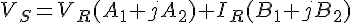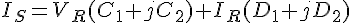where 'S' stands for sending-end and 'R' stands for receiving-end

(a) Given the parameter values in TABLE C and an open-circuit received voltage measured as 88.9 kV, calculate the values of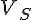and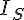and hence the power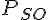absorbed from the supply by the transmission line on open circuit.

(b) If the line is modeled by the T-circuit of FIGURE 3(b), see if you can estimate the primary line coefficients R, L, G and C. The line is 50 km long.

## Homework Equations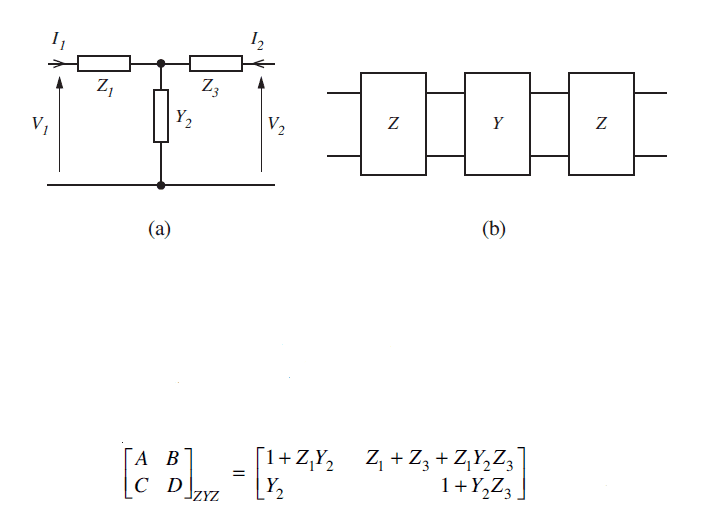## The Attempt at a Solution

#### Attachments

Last edited by a moderator:
Regarding question b) Do I need to go down the route of Z1=Z3=1/2(R+j omega L) x length
and Y2 = (G + 1 omega C) x length ? any help appreciated...

And then form matrix [ A B ]
[ C D ] ?

If the xmsn line is open-circuited at R, what are VR and IR?

And to get PSO, what is the expression for the input impedance of an unterminated ABCD network? Combine the answer for that with the answer to the previous question to get the answer to this question.

This question has got me baffled, so if anyone could point me in the right direction, it would be much appreciated.

With question (a)

If the receiving end is open circuit Ir=0, therefore the equation becomes:

Vs=Vr(A1+jA2)

Vs=88900(0.8698+j0.03542)

Vs= 77325.22+j3148.84

Is=Vr(C1+jC2)

Is=88900(0+j0.001349)

Is=j119.93

Now the power should be calculated by

P=Real{VsIs*}

P=Real{(77325.22+j3148.84)(j119.93)}

P=377565w

I'm hoping I'm on the right track with this?

This problem may be unsolvable. The receiving voltage VR's magnitude is given but not its phase. Was it intended that the phase be zero?

Hi RM

I'm not sure to be honest, but the question does only say estimate. I've been reading through various textbooks, but cannot find any similar questions to this. I'm trying to form an ABCD matrix from the lumped components.

Initially I was concerned that my Vs was incorrect due to it being lower than Vr, but after researching open ended transmission lines, this appeared correct. How do my figures look? That's quite a bit of power consumed @ 37.8 kW, but it is however 50 km in length.

Any pointers?

Electest said:
Hi RM
EDIT: I'm finally looking seriously at your first post and the work you did, and most of it looks very good. Stand by for my answer to your first post.

I'm trying to form an ABCD matrix from the lumped components.
You already have the ABCD parameters, both numerically (your table) and in relation to the T network Z1, Z3 and Y1.

For part (b), as I said you already have the relations between the ABCD parameters and T-network components, so just solve for Z1, Z3 and Y3 from those.
Initially I was concerned that my Vs was incorrect due to it being lower than Vr, but after researching open ended transmission lines, this appeared correct. How do my figures look? That's quite a bit of power consumed @ 37.8 kW, but it is however 50 km in length.
I will compute power myself a bit later and tell you qualitatively how close your answer is to mine.

Last edited:
Electest said:
This question has got me baffled, so if anyone could point me in the right direction, it would be much appreciated.

With question (a)

If the receiving end is open circuit Ir=0, therefore the equation becomes:

Vs=Vr(A1+jA2)

Vs=88900(0.8698+j0.03542)

Vs= 77325.22+j3148.84

Is=Vr(C1+jC2)

Is=88900(0+j0.001349)

Is=j119.93
Up to here all looks 100% unless you made a math error.
Now the power should be calculated by
P=Real{VsIs*}
Real power is (1/2)(VsIs* + Vs*Is). Vs is complex since we've defined Vr as having zero phase. P is a real number.
Repeat your computation of P using this formula.
see my penultimate post for part (b)

Hi RM

I recalculated the power absorbed to get an answer of 377590W. My textbooks only showed the formula I used above, where * indicates the complex conjugate. I've been trying to find reference to the formula you provided which extended this, but could not find any. Could you possibly point me in the direction of a reference as I'm keen to learn. So I was slightly out with my initial figure.

Thanks

Electest said:
Hi RM

I recalculated the power absorbed to get an answer of 377590W. My textbooks only showed the formula I used above, where * indicates the complex conjugate. I've been trying to find reference to the formula you provided which extended this, but could not find any. Could you possibly point me in the direction of a reference as I'm keen to learn. So I was slightly out with my initial figure.

Thanks
You did very well! I apologize for not paying closer attention to your work earlier.
And guess what - our two formulas are identical! Which I found out after some simple math.
So you should have gotten the same answer: P = VsrIsr + VsiIsi.
where r means real part and i means imaginary part.
EDIT: note I changed the expression for P (sign change from last post).

PS - looked at part (b) yet?

Last edited:
rude man said:
You already have the ABCD parameters, both numerically (your table) and in relation to the T network Z1, Z3 and Y1.

For part (b), as I said you already have the relations between the ABCD parameters and T-network components, so just solve for Z1, Z3 and Y3 from those.

In the table we had A1, B1, C1, D1 and A2, B2, C2, D2 - would i be correct in thinking that:

A1...B1...=...0.8698...47.94
C1...D1...0....0.8698

represents the series impedance - Z1 in the T circuit in the first post and that:

A2...B2...=...0.03542...180.8
C2...D2.....0.001349...0.03542

represents the shunt admittance - Y2 in the T circuit?

So to get ABCD matrix for the ZYZ circuit i need to multiply the 3 together to get a single matrix?

If that's the case i have the single matrix to be:

0.083......142.84
0.00102.....0.083

but then i can't get that to tally with

1+Z1Y1....Z1+Z2+(Z1Y2Z3)
Y2......1+Y2Z3

Am i going about this in the correct way or am i totally off-course? Also apologies for the formatting, i don't know all of the tags to space correctly.

Last edited:
Gremlin said:
In the table we had A1, B1, C1, D1 and A2, B2, C2, D2 - would i be correct in thinking that:

A1...B1...=...0.8698...47.94
C1...D1...0....0.8698

represents the series impedance - Z1 in the T circuit in the first post and that:

A2...B2...=...0.03542...180.8
C2...D2.....0.001349...0.03542

represents the shunt admittance - Y2 in the T circuit?

Or if my assumption above is correct, looking at my notes, Z1=47.94 & Y2=0.001349

Last edited:
@Gremlin, you can solve for Z1, Y2 and Z3 in terms of the ABCD parameters.
There are only 4 ABCD parameters:
A1 + jA2,
B1 + jB2,
C1 + jC2,
D1 + jD2.
You are not to separate real and imaginary parts of the four parameters the way you tried to do. You should solve for the three T-equivalent parameters, each of which will (probably) be complex. From that T-network you can then compute the power furnished by the source.
Look some more at posts ## 9 and 11.
EDIT: you can use the T-equivalent network as given in fig. 3(b) of the OP's post 1. The series resistors are R, the parallel resistor is 1/G, and the L and C are obvious.

Last edited:
Thanks rude man,

Just so we don't get our wires crossed here I've already completed part a) and my posts #12 & #13 relate to part b) - don't your posts #9 & #11 relate to part a)?

Ok I've got Z1, Y2 & Z3.

rude man said:
[EDIT: you can use the T-equivalent network as given in fig. 3(b) of the OP's post 1. The series resistors are R, the parallel resistor is 1/G, and the L and C are obvious.

Once you have the impedance how do you go about splitting them into L & R? And the same with splitting Y2 into G & C? You say it's obvious, which slightly unnerves me as it isn't obvious to me at the moment.

Z1 = 0.5 (R +jωL) x length of line.
Z1 = 26.26 + j96.52
f = 50
l = 50km

26.26 + j96.52 = 0.5 (R + j 2*pi*50 L) * 50

Not sure from that a) how you can isolate R or L and b) whether it should be 50km or 50000m

Similarly
Y2 = (G + jωC) x length of line.

Gremlin said:
Once you have the impedance how do you go about splitting them into L & R? And the same with splitting Y2 into G & C? You say it's obvious, which slightly unnerves me as it isn't obvious to me at the moment.

Z1 = 0.5 (R +jωL) x length of line.
Z1 = 26.26 + j96.52
f = 50
l = 50km

26.26 + j96.52 = 0.5 (R + j 2*pi*50 L) * 50

.

After a bit of thought and following this equation through:

(26.26 + j 96.52) x 2 = (R + j 2*pi*50*L) * 50

(52.5128 + j 193.0318) / 50 = R + j 2*pi*50*L

1.05 + j 3.86 = R + j 2*pi*50*L

R = 1.05Ω per km
L = j 3.86 / j 2*pi*50 = 0.0122 H per km

Does that look like the right way of doing it?

Gremlin said:
Once you have the impedance how do you go about splitting them into L & R? And the same with splitting Y2 into G & C? You say it's obvious, which slightly unnerves me as it isn't obvious to me at the moment.

Z1 = 0.5 (R +jωL) x length of line.
Z1 = 26.26 + j96.52
f = 50
l = 50km

26.26 + j96.52 = 0.5 (R + j 2*pi*50 L) * 50

Not sure from that a) how you can isolate R or L and b) whether it should be 50km or 50000m

Similarly
Y2 = (G + jωC) x length of line.
Z1 = R + jwL = Z3
Y2 = G + jwC
Each impedance/admittance has a real and an imaginary part. The relationship with R,L,C and G should be obvious once you've computed Z1, Z3 and Y2 in terms of A,B,C and D.
BTW don't mix up C of the ABCD's with the line capacitance C.
The length of the line is implied in the ABCD parameters. You should have Z1, Z3 and Y2 strictly in terms of the complex ABCD parameters. You have that relationship given to you, albeit indirectly (cf. your post 1, section 2)..
Remember f = 50 Hz.
Hint: since the line is unterminated, does Z3 matter at all? You can make life much simpler by deleting Z3. Your VR voltage is then measured at the mid-point of the original T-equivalent network.
One thing: they ask for R,L.G and C. Usually, these parameters are per unit length of line. Since they don't give you the length of the line you have to assume R,L,C and G are for the WHOLE line. That is unusual and misleading IMO.
Gremlin said:
Thanks rude man,
Just so we don't get our wires crossed here I've already completed part a) and my posts #12 & #13 relate to part b) - don't your posts #9 & #11 relate to part a)?
Yes. Post 14 and this one are the only ones addressing part (b).

Gremlin said:
After a bit of thought and following this equation through:

(26.26 + j 96.52) x 2 = (R + j 2*pi*50*L) * 50

(52.5128 + j 193.0318) / 50 = R + j 2*pi*50*L

1.05 + j 3.86 = R + j 2*pi*50*L

R = 1.05Ω per km
L = j 3.86 / j 2*pi*50 = 0.0122 H per km

Does that look like the right way of doing it?
The answers are not "per km" unless the line is given as 1 km long. Are you using SI units faithfully? I can't check all your math, sorry.

rude man said:
The answers are not "per km" unless the line is given as 1 km long. Are you using SI units faithfully? I can't check all your math, sorry.

It's states in part b) of the question that the line is 50km long.

In post #18 i divided the impedance by 50 because of this to give me a per km answer.

Gremlin said:
It's states in part b) of the question that the line is 50km long.

In post #18 i divided the impedance by 50 because of this to give me a per km answer.
Right! Sorry, I missed that. OK, the ABCD parameters are for the whole line, though, so your T network also represents the whole line. So Z1, Z3 and Y2 also represent the whole line.So you are right to divide all four of R,L,C and G by 50 to get per km.

If you come up with a numerical answer for part (b) I will tell you if it's what I got. I mean, if you finish with G and C. I see you already have R and L.

Sorry, I have to retract my promise to check your R,L,C and G. I'd have to do the same math you did. I thought I could "cheat" past that too but no. But that should be just basic algebra (use Wolfram Alpha to solve for Z1 and Y2 in terms of A,B,C and D?).
I think you're there anyway.

Gremlin said:
Once you have the impedance how do you go about splitting them into L & R? And the same with splitting Y2 into G & C? You say it's obvious, which slightly unnerves me as it isn't obvious to me at the moment.

Z1 = 0.5 (R +jωL) x length of line.
Z1 = 26.26 + j96.52
f = 50
l = 50km

26.26 + j96.52 = 0.5 (R + j 2*pi*50 L) * 50

Not sure from that a) how you can isolate R or L and b) whether it should be 50km or 50000m

Similarly
Y2 = (G + jωC) x length of line.

Hi just wondering how you got to have Z1 = 26.26 + j96.52

I did Z1 = VS / IS

I'm getting Z1 = 26.26 - j644.75

What am I doing wrong?

dear Help,

I m trying to calculate the power:

when using the formula P=1/2Re(VI*) 1/2RE(77325.2+j3148.84)(j119.926= -188814
but when i use

P=VsrIsr+VsiIsi = 77325.2+(j3148.84xj119.926)= -300303

where do i go wrong?

Am I correct in saying that Y2 is fairly easy to find?

If using the ABCD parameters given and using the parameters for a cascaded ZYZ matrix then Y2 is equal to parameter C in this case?

From there I would rearrange from the ZYZ parameters, 1 + Z1Y2, to get Z1.

Is this a correct method to find Z1 and Y2 or am I way off track?

Any help will be greatly appreciated! :-)

Consider a general ABCD matrix:

[ A B ]
[ C D ]

Now if you have a T network that has that matrix, the elements of the T network will be:

Left series element = (A-1)/C

Shunt element = 1/C

Right series element = (D-1)/C

You can work this out by considering how the ABCD matrix is formed from a T network.

Note that the numerical value your problem gave you for C is 0 + .001349 j

This means that G is zero, which is what I suggested you assume when solving those equations above.

But, now if the shunt element of the T network is 1/C , we just need to solve (because G=0):

1/C = 1/(.001349 j) = 1/(j ω Cs), where Cs (I designate it Cs instead of just C to avoid confusion with the C of the matrix) is the shunt capacitance of the T network.

R/2 will be the real part of (.8698 + .03542 j - 1)/(.001349 j)

and L/2 will be the imaginary part of (.8698 + .03542 j - 1)/(.001349 j) divided by ω.

These values of R, L and Cs are for the whole line; divide by the line length for the final result.

I am also doing this question and am confused by part b. Can this be solved with simultaneous equations ? my conundrum is that I will have 4 terms and want 3 results

Thunderchild said:
I am also doing this question and am confused by part b. Can this be solved with simultaneous equations ? my conundrum is that I will have 4 terms and want 3 results

Read the section on ABCD parameters here: https://en.wikipedia.org/wiki/Two-port_network

Especially note that for symmetric networks, A=D. For this problem, symmetry means that Z1=Z3, so you only need 2 results.